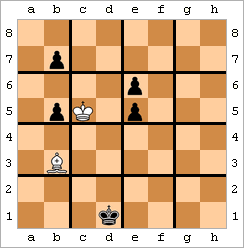# Two Simple Math Puzzles: Prime Numbers and Shortest Path

Posted on:June 20, 2015 at 12:00 AM

Here are two math puzzles, solve, comment and enjoy the discussion!

## Puzzle 1: Prime NumbersSection titled Puzzle 1: Prime Numbers

Prove that $p^2-1$ is divisible by 24, where $p$ is a prime number with $p>3$.

This is a simple one - do not go by the technicality of the problem statement.

### Solution 1Section titled Solution 1

$p^2-1 = (p-1)\times (p+1)$ Given, $p$ is a prime number $>3$, $p-1$ and $p+1$ are even. We can also write,

\begin{aligned} p-1=2K, \text{and } \cr p+1=2K+2=2(K+1) \end{aligned}

Given, $K \in \mathbb{N}$, either $K$ or $K+1$ are also even. Hence, $(p-1)\times (p+1)$ is divisible by $2\times 4 = 8$.

Furthermore, as $p$ is prime, we can write it as either $p = 3s+1$ or $p = 3s-1$, where $s \in \mathbb{N}$. In either case, one of $p-1$ or $p+1$ are divisible by 3 as well.

Hence, $p^2-1$ is divisible by $8\times 3 = 24$.

## Puzzle 2: Hopping on a Chess BoardSection titled Puzzle 2: Hopping on a Chess Board

On a chess board, basically a $8\times 8$ grid, how many ways one can go from the left most bottom corner to the right most upper corner? In chess naming conventions, from $a1$ to $h8$.Can you find the generic answer for the case of $N\times M$ grid?

### Solution 2Section titled Solution 2

Given only forward moves are allowed, for any arbitrary grid of $N\times M$, a total of $(N-1) + (M-1)$ moves are needed.

Any $N-1$ of these moves can be of type right and $M-1$ of type up. In short, this is a combinatorial problem of distributing $N+M-2$ objects into groups of $N-1$ and $M-1$. This is simply,

$\dbinom{N+M-2}{N-1} = \frac{(N+M-2)!}{(N-1)! (M-1)!}$

In the particular case of the chess board, $N = M = 8$. Hence, total number of possible paths are:

$\text{No. of Paths} = \frac{14!}{7! 7!} = 3432$

Let me know if you have any other interesting alternative solutions to these problems.